2037.0.30.001 - Microdata: Census of Population and Housing, Census Sample File, 2011
ARCHIVED ISSUE Released at 11:30 AM (CANBERRA TIME) 12/12/2013
 Page tools: .mffeedback,#pjs { display :none; } Enable Javascript to Print PagesPrint AllEmail Notification This document was added or updated on 13/12/2013. METHODOLOGY SELECTION OF SAMPLE Data in the Census Sample Files (CSFs) represent 1% and 5% samples of all dwelling, family and person records from the 2011 Census of Population and Housing. The 1% Basic CSF provides a sample of one private dwelling record in every hundred from the Census, and the associated family and person records. Dwellings with more than six usual residents were removed from the sample to ensure confidentiality of large dwellings (see Large households). For non-private dwellings the sampling is applied to persons present, where one person in every hundred is selected and the associated dwelling records included on the file. The 5% Expanded CSF provides a sample of one private dwelling in every twenty from the Census, and the associated family and person records. Dwellings with more than eight usual residents were removed from the sample to ensure confidentiality of large dwellings (see Large households). For non-private dwellings the sampling is applied to persons present, where five persons in every hundred are selected and the associated dwelling records included on the file. The 1% CSF and the 5% CSF also contain corresponding family and person records for the selected private dwellings, as well as a 1 in 100 (or 1 in 20) sample of person records from non-private dwellings. Person, family and dwelling estimates can be obtained from the CSF for private dwellings, but only person level estimates are available for non-private dwellings, and hence for the whole Census population. Large households To ensure the confidentiality of large households in occupied private dwellings, the number of persons for each household is restricted to a maximum of six usual residents on the 1% Basic CSF and eight usual residents on the 5% Expanded CSF. Dwellings with more than six usual residents for the 1% Basic CSF, or eight usual residents for the 5% Expanded CSF, have been replaced by dwellings of a similar size and from a similar region that do not have more than the maximum number of usual residents. Persons in Other Territories, comprising Jervis Bay, Cocos (Keeling) and Christmas Islands, have been excluded from the sample, as have migratory, shipping and off-shore statistical areas. Changes in previous CURFs can be found in Changes from previous CSF. ESTIMATION PROCEDURE An estimate of the total for an item can be obtained by totalling the item for the CSF and then multiplying the result by 100 for the 1% CSF, or by 20 for the 5% CSF. Note that this estimate of total will not correspond exactly to the total that would be obtained from the full Census, firstly because of the exclusion of large dwellings from the CSF, and secondly because of the sampling error arising due to the CSF containing only a sample of Census records. Averages from the CSF, such as the proportion of persons falling into a particular category, can be used as an estimate of the corresponding average in the Census. For example, the proportion of Australian-born persons who are students is estimated by the proportion of students observed among Australian-born persons on the CSF. Note that if the denominator of such a proportion is known from the full Census then it can be multiplied by the estimated proportion to give an estimate of the numerator. For example, the total number of Australian-born students could be estimated by multiplying the above proportion by the Australian-born population. This gives an alternative estimate from the CSF (rather than counting the Australian-born students on the 1% CSF and multiplying by 100) that may be preferred in some circumstances, since it is more compatible with the known full-Census count. RELIABILITY OF ESTIMATES The sampling error should be taken into account when interpreting estimates from the CSF. A measure of the likely difference between an estimate from the CSF and the corresponding full Census value is given by the standard error (SE) of the estimate. The SE indicates the extent to which an estimate might have varied by chance because only a sample of persons was included. There are about two chances in three that a sample estimate will differ by less than one SE from the full Census value, and about 19 chances in 20 that the difference will be less than two SEs. Another measure of sampling variability is the relative standard error (RSE) which is obtained by expressing the SE as a percentage of the estimate to which it refers. Non-sampling errors may occur in any enumeration - a full count or a sample - and should not be confused with imprecision due to sampling error, which is measured by the SE. Non-sampling errors in the CSF are differences due to the exclusion of large dwellings, while in the Census as a whole there may be inaccuracies that occur because of imperfections in reporting by respondents, errors made in collection (such as when recording responses) and errors made in processing the Census data. It is not possible to quantify non-sampling error, but every effort is made to reduce it to a minimum. For the following examples, non-sampling error is assumed to be zero. In practice, the potential for non-sampling error adds to the uncertainty in the estimates that is caused by sampling variability. Standard error calculation Both CSFs can be treated, for the purposes of standard error calculations, as a simple random sample of dwellings from the private dwelling population. For many purposes the non-private dwelling population has only a minor influence on results, and it is sufficient to include each person counted in a non-private dwelling as a separate 'dwelling' when calculating standard errors. Dwelling level estimates Estimates of the SE of averages for dwelling-level items can be obtained using standard formulae for a simple random sample. These standard error formulae require computing the average value of an item of interest per dwelling on the CSF. The formula for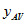, the estimated average of an item that takes value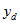for dwelling d out of n sampled dwellings in a geographic area, is: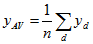where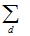represents summing over the n dwellings. The standard error estimate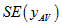is given by the following formula: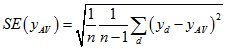The estimate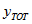of the total count for this item, and its corresponding SE estimate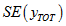, are obtained by multiplying the average per dwelling by the number of dwellings in the geographic area. The number of dwellings is approximated with minimal error by: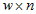where w is the weight (100 on the 1% CSF, and 20 on the 5% CSF) since the construction of the CSF ensures proportional representation of geographic areas. The formulae are as follows: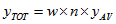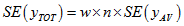Note that the geographic area to be used in these calculations should be the smallest geographic area containing the dwellings in question. For example, estimates for a single state should use state as the geographic area. Person level estimates The above formulae can be applied to totals of persons by treating theas person counts within the dwelling i.e.is the number of persons from dwelling d with the characteristic of interest. This makesthe average number of persons per dwelling having this characteristic, andthe total number of persons in the geographic area with this characteristic. Family level estimates Similarly, estimates for family-level items can be obtained by treating theas family counts within the dwelling i.e.is the number of families from dwelling d with the characteristic of interest,is the average number of families per dwelling having the characteristic, andis the total number of families in the geographic area with the characteristic. Example of standard error calculation The Australian-born population of Australia from the 2011 Census is 15,017,845. As the CSF is based on place of enumeration counts, this figure can be obtained from the Place of Enumeration Profile for Australia on the ABS website. However, as the CSF excludes Other Territories, 1,728 Australian-born persons enumerated in Other Territories on Census Night should be excluded, giving a total of 15,016,117 Australian-born persons. Note that persons enumerated in shipping, migratory or off-shore statistical areas are also excluded from the CSF, but as these counts should be minor, and are not easily accessible from the ABS website, they have been ignored from the calculations. The 1% CSF estimate of this figure is calculated by taking the 149,324 Australian-born persons on the 1% CSF and multiplying it by 100, giving an estimate of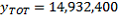. The difference between this figure and the full Census figure of 15,016,117 is due to both the exclusion of large dwellings from the CSF and also the sampling error of the CSF estimate. Note that similar calculations can be carried out using data from the 5% CSF, but using a weight of 20. For simplicity, the remaining examples will be based on the 1% CSF figures. The simplest way to calculate the SE of this estimate is to produce a file with a single record for each dwelling (treating each person from non-private dwellings as a separate dwelling). On this file, the itemshould give the number of Australian-born persons in the dwelling. A simple aggregation can be applied to this file to calculate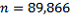(the count of dwelling records),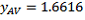(the mean) and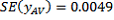(the standard error of the mean). These are then used to estimate the total and its SE: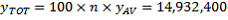(as calculated previously), and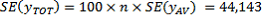This SE calculation suggests that there are about two chances in three that the sample estimate will differ by less than 44,143. from the full-Census value, and about 19 chances in 20 that the difference will be less than 2 x44,143 = 88,286 . The range (14,932,400 - 88,286; 14,932,400 + 88,286) = ( 14,844,114; 15,020,686) is known as the 95% confidence interval. In this example the Census value of 15,016,117 lies within the confidence interval range. The estimate is low because the CSF excludes some 1,523 persons from large dwellings, approximately 69% of whom would have been born in Australia. This corresponds to excluding approximately 1,523 x 100 x 69% = 105,087 persons from the estimate. Ignoring the effect that these extra people would have had on the standard error, they would increase the estimate to approximately 15,037,487 Australian-born persons and the 95% confidence interval to (15,037,487 - 88,286; 15,037,487+ 88,286) = ( 14,949,201; 15,125,773). Whilst the initial confidence interval just covers the true value, the revised confidence interval more comfortably covers the true population value of 15,016,117. Users may wish to reproduce these figures using the CSF to ensure that they have interpreted the calculations required correctly. Clustering of the person sample For some person-level variables, it may be a reasonable approximation to treat the CSF as a simple random sample of persons, even though it is in fact a sample of dwellings. This would involve letting d in the above formulae indicate persons rather than dwellings, and replacing n by the number of persons in the CSF geographic area of interest. Person means and associated standard errors could then be obtained by a standard tabulation package applied to the person-level data. Unfortunately, doing this will typically give an underestimate of the actual SE. The extent of this underestimation depends on how clustered the variable of interest is within dwellings - that is, on how often similar values of the variable tend to occur together in the same dwelling. The understatement of standard error will be greatest for variables that are highly clustered within dwellings, such as birthplace. For this reason it would be appropriate, when treating the CSF as a sample of persons, to obtain a measure of the effect of clustering for the variables being investigated. A suitable measure is the design factor (DEFT), given by the ratio of the SE calculated correctly (with dwellings as units) to the SE calculated treating persons as units. Standard errors from the person-level analysis can then be adjusted by this factor. The SE ignoring clustering will be denoted by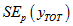, with the subscript p indicating that it is calculated at the person level. This can be obtained by taking the person-level CSF and creating a variable taking the value 1 for Australian-born persons and 0 otherwise. Applying a simple tabulation package to this person-level file gives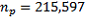(the count of person records),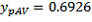(the mean) and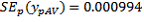(the standard error of the mean). These are then used to estimate the total and its SE.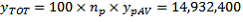(as calculated previously) and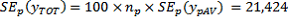The design factor is then given as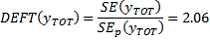Thus the standard error produced ignoring clustering underestimates the actual standard error by a factor of 2. Users could expect that other totals (eg. for geographic regions) for the variable 'Australian-born' would have a similar design factor. Standard errors for proportions and differences Proportions Simple approximations can be used to estimate the standard error for a ratio of counts. If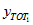and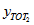are estimated totals for two nested categories (i.e. category 2 is a subset of category 1) then writing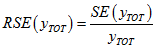for the relative standard error gives the following approximation: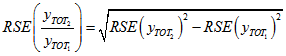This formula depends on the two categories being nested, and should not be used for distinct categories. Differences If two totals are for distinct categories (eg. in comparing estimates across states) then the difference between two totals has the following SE approximation: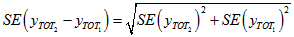While this formula will only be exact for differences between separate and uncorrelated (unrelated) characteristics or sub-populations, it is expected to provide a good approximation for most differences likely to be of interest. Example of a standard error calculation for a proportion The number of Australian-born persons who are full-time students (STUP=2) can be estimated by producing a dwelling-level file with a variable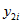giving the number of Australian-born full-time students in the dwelling. Tabulating this variable gives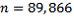,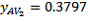,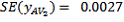, so that: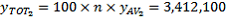, and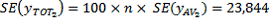Thus there are an estimated 3,412,100 Australian-born full-time students, with a SE of 23,844 and an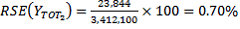. The RSE of the estimate of Australian-born persons is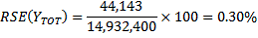(see earlier calculations). Thus the estimated proportion of Australian-born persons who are full-time students is given by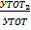=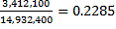. The RSE of this proportion is estimated as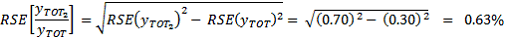Thus, the RSE on the estimated proportion of 0.2285 is 0.63% and hence the SE is 0.0014. Regression estimates One use of the sample file will be to examine relationships between variables using regression methods. By treating the dwelling as the sample unit, standard regression packages can be used unweighted and the resulting standard errors and test statistics will be good estimates. For example, a regression model could be derived for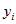, the number of persons in the dwelling needing assistance with core activities, against various characteristics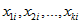Equation such as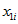, the number of persons in the dwelling aged over 65 years, to fit the linear regression model: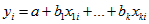Measures of model fit and of significance of the parameters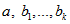from the standard package will then be appropriate. Unfortunately such a linear model may not adequately describe the relationships between variables at a dwelling level. If a similar regression is performed treating person as the sample unit, the resulting standard errors and measures of significance could be inaccurate or misleading. This arises because the persons in the sample are clustered within dwellings, and so their responses may be "correlated" or affected by similar influences such as characteristics of the dwelling. The extent to which the measures of significance are affected will depend on how clustered the variableis likely to be within dwellings. If a person-level analysis is performed, such as a 'logistic analysis' of the probability of a person having a given characteristic, then the effect of clustering should be taken into account when interpreting the outcomes. In particular, SEs are likely to be understated, as discussed in the section Clustering of the person sample, and this will tend to increase the apparent significance of modelled effects. Techniques are available to perform valid analyses at the person level for a sample that is clustered within dwellings, treating persons as being subject to both person and dwelling effects. These techniques include 'multi-level', 'random effect' and 'mixed' modelling. (Footnote 1 and 2) By using these techniques, models can be used that do a better job of describing the actual relationships between variables at both person and dwelling level. Statistical packages are widely available to validly perform such analyses. ___________________________ Footnote 1 Goldstein, H. and Arnold, E, 1995, 'Multilevel Statistical Models', 2nd ed.Halsted Press, New York. Footnote 2 Snijders Tom A. B. and Bosker Roel J, 1999, 'Multilevel analysis : an introduction to basic and advanced multilevel modelling, SAGE, London. Document Selection These documents will be presented in a new window.